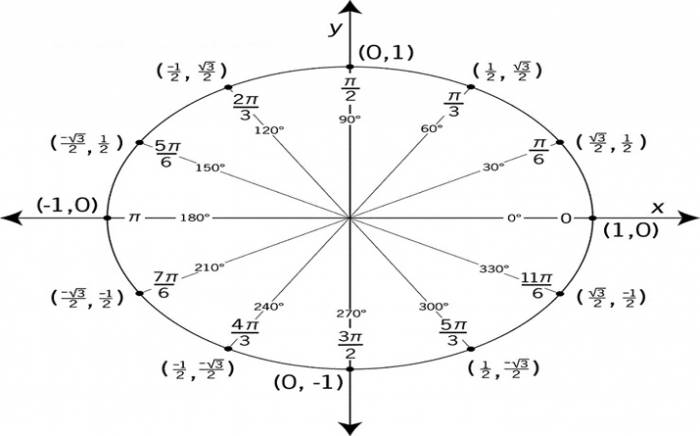The point of the unit circle is that it makes other parts of the mathematics easier and neater. For instance, in the unit circle, for any angle θ, the trig values for sine and cosine are clearly nothing more than sin(θ) = y and cos(θ) = x. Working from this, you can take the fact that the tangent is defined as being tan(θ) = y/x, and then substitute for x and y to easily prove that the value of tan(θ) also must be equal to the ratio sin(θ)/cos(θ).Another thing you can see from the unit circle is that the values of sine and cosine will never be more than 1 or less than –1, since x and y never take on values outside of this interval. Also, since tangent involves dividing by x, and since x = 0 when you’re one-fourth and three-fourths of the way around the circle (that is, when you’re at 90° and at 270°), the tangent will not be defined for these angle measures.

Certain angles have “nice” trig values. These angles, in the first quadrant (being the “reference” angles) are 0°, 30°, 45°, 60°, and 90°. (Strictly speaking, 0deg and 90deg aren’t “in” any quadrant, but we’ll work with them as though they were in the first quadrant. It’s just easier that way.) So you’ll probably be expected to have memorized the trig-function values for these angles. You’ll probably now also be provided with a circle, having those angles marked. In the first quadrant, we have the following:

Any point on the unit circle will be a distance of one unit from the center; this is the definition of the unit circle. To “confirm” that the point they’ve given me is a point on the unit circle, I can apply the Pythagorean Theorem to find the length of the radius of the right triangle formed by dropping a perpendicular from the x-axis down to the point. My perpendicular is the bright blue dashed line. If you want to learn trigonometry you can get good information from trigonometric identities.

If the Pythagorean Theorem gives me a value for the radius of 1, then I’ll have “confirmed” that the point is on the unit circle.